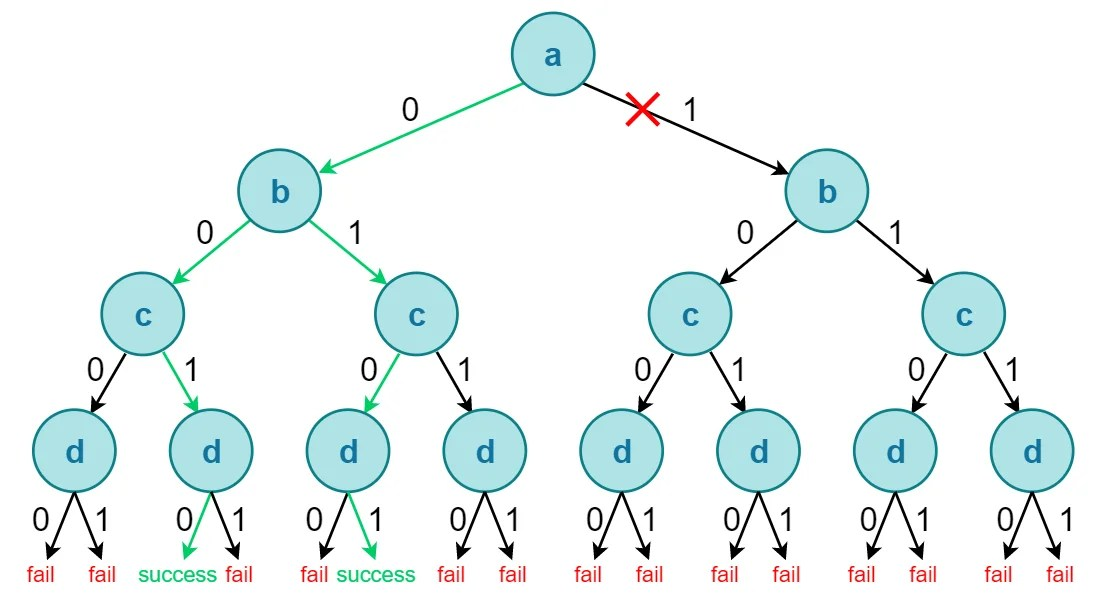View Course Path

# Automatic Test Pattern Generation (ATPG) in DFT (VLSI)

Test pattern generation (TPG) is the process of generating test patterns for a given fault model. If we go by exhaustive testing, in the worst case, we may require 2n (where n stands for no. of primary inputs) assignments to be applied for finding test vector for a single stuck-at fault. It is impossible for us to manually use exhaustive testing or path sensitization method to generate a test pattern for chips consisting of millions of transistors. Hence, we need an automated process, a.k.a. Automatic Test Pattern Generation (ATPG).

Automatic Test Pattern Generation, or ATPG, is a process used in semiconductor electronic device testing wherein the test patterns required to check a device for faults are automatically generated by a program.

Contents

## ATPG Software

ATPG is a decision problem. Consider a combinational circuit with four primary inputs (a, b, c, d). You’ll find below a binary decision tree formed out of a truth-table showing all possible input vectors. In a binary decision tree, at every level, we have two decisions (either assign logic-0 or logic-1). This tree consists of 16 different possible vectors. The solution can be found at the bottom of the tree. There could be more than one or no solutions too. To search the test vector required for the given fault, an equivalent algorithm can be written in high-level languages like C++ or Python.Adding another primary input e would double the size of the tree. The decision tree size is exponential to the number of primary inputs! Hence, we need smart heuristics like branch pruning to be embedded in the algorithm to speed up the process. The red cross in the above diagram is the result of pruning since there are no solutions available below this branch.

The software used for complex ATPG applications are quite expensive, but the process of generating a test needs to be done only once at the end of the design process.

## ATPG classification

### Based on Algorithm

There are two types of ATPG algorithms:

 Complete ATPG Incomplete ATPG Traverses through the whole search tree, which is exponential in size. Does not exhaust the whole search tree. If a test-vector exists at the bottom, then the algorithm will find it for sure. May not guarantee to find a solution even if it exists. Consumes more CPU time. Consumes lesser CPU time.

Hence, there is a trade-off between test pattern generation time and fault coverage between the two algorithms.

### Based on Application

As we have frequently noticed in this DFT course, DFT techniques and test generation become significantly tricky for sequential circuits as compared to combinational circuits. This is due to controllability and observability issues in internal flip-flops and latches. Hence, the ATPG algorithms are applied separately for combinational and sequential circuits. Based on application, the ATPG algorithm is broadly classified into two types:

• Combinational ATPG (e.g. D, PODEM, FAN)
• Sequential ATPG (e.g. Extended D, 9-valued)

These methods are only applicable for single stuck-at faults. Pattern generation through any of these algorithmic methods requires what is known as the path sensitization method. Most algorithmic generation methods also refer to notations D and D’.  These notations were introduced by the D algorithm and have been adopted by other algorithms since then.

## Stages of ATPG

ATPG algorithm is a two-stage process.

Random Test Pattern Generation: In this method, we randomly generate test patterns and select those patterns that detect undetected faults. There is no target fault. Since test patterns are generated by trial and error method, this is a pretty fast and inexpensive process. It’s just like throwing darts being blindfolded with lots and lots of targets around. Random TPG is also known as Probabilistic ATPG.

Deterministic Test Pattern Generation: In this method, we select a specific target fault and apply various algorithms like D, PODEM, or FAN. This is a time-consuming algorithmic method and relatively expensive than Random TPG.

The idea of Random TPG is that there are many easy-to-detect faults. Hence, we run Random TPG first, which is a speedy process as compared to time-consuming Deterministic TPG. The problem with Random TPG is that fault coverage saturates after the easy faults are detected. Deterministic TPG is only used for those faults which are undetected in Random TPG. These faults are also known as random pattern resistant faults.The effectiveness of the ATPG is measured primarily by the fault coverage achieved and the cost of performing the test (algorithm complexity and test length).

## Benefits of ATPG

The following are the benefits of ATPG that made it popular in the EDA industry.

• Generates high-coverage test patterns
• Lowers test time and cost
• Reduce Human efforts
• Ensures easy, risk-free deployment into design and test flows

## Summary

In this article, we discussed and introduced ATPG, which clears our pain for test pattern generation in large circuits. However, for computers, ATPG is still a slow and expensive process. Hence, random test pattern generation is performed before time-consuming ATPG algorithms, which is very beneficial in decreasing test time.

In exceptional cases, we may need to incorporate an incomplete ATPG algorithm if adequate fault coverage is possible. Heuristics like branch pruning have proven to be very efficient in test pattern generation too.

In the next few articles, we will do a study of various combinational ATPG algorithms like D, PODEM, and FAN.

Related courses for this will be up soon!

This site uses Akismet to reduce spam. Learn how your comment data is processed.

Top